Courses

# Centre Of Mass Practice Level -1

## 30 Questions MCQ Test | Centre Of Mass Practice Level -1

Description
This mock test of Centre Of Mass Practice Level -1 for Class 11 helps you for every Class 11 entrance exam. This contains 30 Multiple Choice Questions for Class 11 Centre Of Mass Practice Level -1 (mcq) to study with solutions a complete question bank. The solved questions answers in this Centre Of Mass Practice Level -1 quiz give you a good mix of easy questions and tough questions. Class 11 students definitely take this Centre Of Mass Practice Level -1 exercise for a better result in the exam. You can find other Centre Of Mass Practice Level -1 extra questions, long questions & short questions for Class 11 on EduRev as well by searching above.
QUESTION: 1

Solution:
QUESTION: 2

Solution:
QUESTION: 3

### A bullet of mass 0.01 kg and travelling at a speed of500 m/s strikes a block of 2 kg which is suspended bya string of length 5 m. The centre of gravity of the blockis found it rise a vertical distance of 0.1 m. Which isthe speed of the bullet after it emerges from the block?

Solution:
QUESTION: 4

A ball of mass m moving with velocity v strikes the bob of a pendulum at rest. The mass of the bob is also m. If the collision is perfectly inelastic, the height to which the bob will rise is given by

Solution:
QUESTION: 5

If in the previous problem the collision is elastic, the height to which the bob will rise will be

Solution:
QUESTION: 6

The first ball of mass m moving with a velocity u collides head on with the second ball of mass m at rest. If the coefficient of restitution is e, then the ratio of the velocities of the first and the second ball after the collision is

Solution:
QUESTION: 7

A ball impinges directly on another ball at rest. The first ball is brought to rest by the impact. If half of the kinetic energy is lost by the impact, the value of coefficient of restitution is

Solution:
QUESTION: 8

A 20 g bullet pierces through a plate of mass M1 = 1 kg and then comes to rest inside a second plate of mass M2 = 2.98 kg as shown in Fig. It is found that the two plates, initially at rest, now move with equal velocities. Find the percentage loss in the initial velocity of thebullet when it is between M1 and M2. Neglect any loss of material of the plates due to the action of bullet.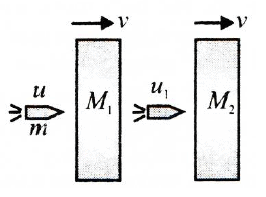Solution:
QUESTION: 9

A particle of mass m is moving horizontally with aconstant velocity v towards a rigid wall that is movingin opposite direction with a constant speed u. Assumingelastic impact between the particle and wall, the workdone by the wall in reflecting the particle is equal to

Solution:
QUESTION: 10

Two identical balls A and B are released from theposition shown in Fig. They collide elastically with eachother on the horizontal portion. The ratio of heightsattained by A and B after collision is (neglect friction)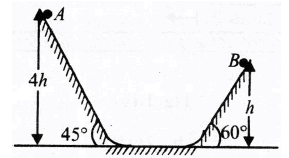Solution:
QUESTION: 11

Block A of mass M = 2 kg is connected to anotherblock B of mass m = 1 kg with a string and a spring offorce constant k = 600 N/m as shown in Fig. . Initially,spring is compressed to 10 cm and whole system ismoving on a smooth surface with a velocity v = 1 m/s.At any time, thread is burnt, the velocity of block A,when B is having maximum velocity w.r.t. ground, is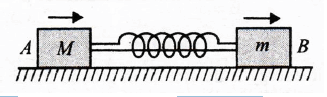Solution:
QUESTION: 12

Two identical blocks, having mass M each, areconjugated and placed on a smooth floor as shown inFig. A small block of mass m is released from positionas shown. Velocity of block B is maximum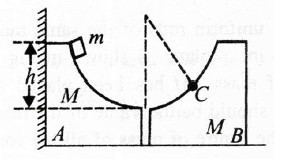Solution:
QUESTION: 13

A particle of mass m travelling with velocity v and kinetic energy E collides elastically to another particle of mass nm, at rest. What is the fraction of total energy retained by the particle of mass m?

Solution:
QUESTION: 14

A ball of mass m moving with speed u undergoes a head-on elastic collision with a ball of mass nm initially at rest. The fraction of the incident energy transferred to the second ball is

Solution:
QUESTION: 15

In the above question, the ratio of the kinetic energies of the ‘first ball to the second ball after collision is

Solution:
QUESTION: 16

The graph between kinetic energy and momentum of aparticle is plotted as shown in Fig. The mass of themoving particle is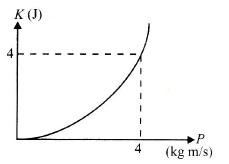Solution:
QUESTION: 17

A trolley is moving horizontally with a velocity of v m/s w.r.t. earth. A man starts running in the direction of motion of trolley from one end of the trolley with a velocity 1.5 v m/s w.r.t. the trolley. After reaching the opposite end, the man turns back and continues running with a velocity of 1.5 v m/s w.r.t. trolley in the backward direction. If the length of the trolley is L, then the displacement of the man with respect to earth, measured as a function of time, will attain a maximum value of

Solution:
QUESTION: 18

Two blocks of masses m and 4m lie on a smoothhorizontal surface connected with a spring in its naturallength. Mass m is given velocity v0 through an impulseas shown in Fig. Which of the following is not trueabout subsequent motion?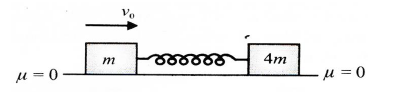Solution:
QUESTION: 19

Three particles A, B and C of equal masses move withequal speeds v along the medians of an equilateraltriangle. They collide at the centroid O of the triangle.After collision A comes to rest while B retraces its pathwith speed v. The velocity of C is then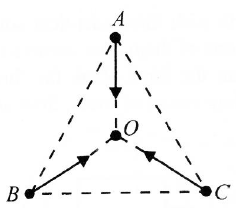Solution:
QUESTION: 20

A body dropped from a tower explodes into two piecesof equal mass in mid-air. Which of the following is notpossible?

Solution:
QUESTION: 21

In perfectly inelastic collisions, the relative velocity ofthe bodies

Solution:
QUESTION: 22

A car of mass 400 kg travelling at 72 km/h crashesinto a truck of mass 4000 kg travelling at 9 km/h in thesame direction. The car bounces back at a speed of18 km/h. The speed of the truck after the impact is.

Solution:
QUESTION: 23

A bomb of mass 12 kg at rest explodes into two piecesof masses 4 kg and 8 kg. The velocity of 8 kg mass is6 m/s. The kinetic energy of the other mass is.

Solution:
QUESTION: 24

A projectile is moving at 20 m s-1 at its highest pointwhere it breaks into equal parts due to an internalexplosion. One part moves vertically up at 30 m s-1with respect to the ground. Then the other part willmove at

Solution:
QUESTION: 25

A body is hanging from a rigid support by an inextensiblestring of length ι . It is struck inelastically by an identicalbody of mass m moving with horizontal velocity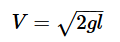, the tension in the string increases justafter striking by

Solution:
QUESTION: 26

Two particles are shown in Fig. At t = 0, a constantforce F = 6 N starts acting on the 3 kg man. Find thevelocity of the centre of mass of these particlesat t = 5 s.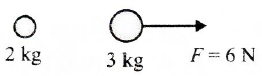Solution:
QUESTION: 27

A U-shaped wire has a semicircular bending betweenA and B as shown in Fig. A bead of mass m movingwith uniform speed v through a wire enters thesemicircular bend at A and leaves at B with velocity v/2 after time T. The average force exerted by the beadon the part AB of the wire is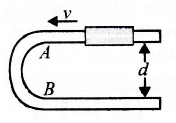Solution:
QUESTION: 28

Two blocks of masses m1 = 2 kg and m2 = 4 kg aremoving in the same direction with speeds v1 = 6 m/sand v2 = 3 m/s, respectively on a frictionless surfaceas shown in figure. An ideal spring with spring constantk = 30000 N/m is attached to the back side of m2Thenthe maximum compression of the spring after collisionwill be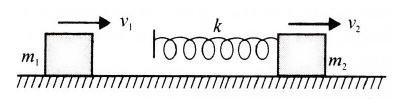Solution:
QUESTION: 29

A force exerts an impulse I on a particle changing itsspeed from initial velocity u to final velocity 2u. Theapplied force and the initial velocity are oppositelyoriented along the same line. The work done by theforce is

Solution:
QUESTION: 30

Determine the y coordinate of centroid of the wire in the shape of circle as shown.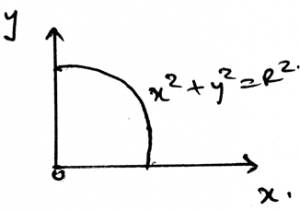Solution:

The weight of the body is the sum of the all the small weights that are been applied by all the particles. The smaller the particle the smaller the weight it applies. Thus the total weight of the body is the summation of all the infinite number of small weights applied to the body.